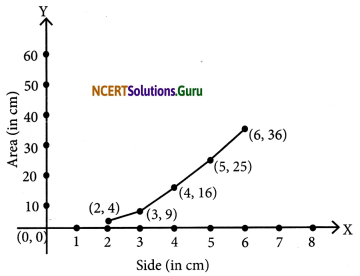# NCERT Solutions for Class 8 Maths Chapter 15 Introduction to Graphs Ex 15.3

These NCERT Solutions for Class 8 Maths Chapter 15 Introduction to Graphs Ex 15.3 Questions and Answers are prepared by our highly skilled subject experts.

## NCERT Solutions for Class 8 Maths Chapter 15 Introduction to Graphs Exercise 15.3

Question 1.
Draw the graphs for the following tables of values, with suitable scales on the axes
(a) Cost of apples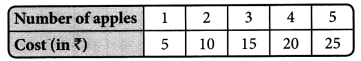(b) Distance travelled by a car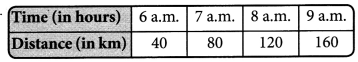(i) How much distance did the car cover during the period 7.30 am to 8a.m.?
(ii) What was the time when the car had covered a distance of 100 km since it’s start?
(c) Interest on deposits for a year.(i) Does the graph pass through the origin?
(ii) Use the graph to find the interest on ? 2500 for a year.
(iii) To get an interest of ₹ 280 per year, how much money should be deposited?
(a) (i) Draw x-axis and y-axis mutually perpendicular to each other.
(ii) Take a suitable scale (x-axis; 1cm = 1 unit) (y-axis; 1cm = 5 units)
(iii) Take the number of apples along the x-axis and mark the cost in ₹ along the y-axis.
(iv) Plot the points (1,5), (2,10); (3,15): (4, 20) and (5,25)
(v) On joining the points, we obtain the graph as a straight line.(b) (i) Draw x-axis and y-axis mutually perpendicular to each other.
(ii) Choose suitable scale along x-axis and y-axis (x-axis; lcm = 1 unit; y-axis; lcm = 20 units.
(iii) Mark time (in hours) along x-axis and distance (in km) along y-axis
(iv) Plot the points (6, 40), (7, 80) (8, 120) and (9, 160)
(v) By joining the points, we get the required graph.
1. In the graph, draw a perpendic-ular at the point indicating 7:30 a.m. on the x-axis such that it meets the graph at A. From A draw a line parallel to x-axis to meet the y-axis at 100 km.
Distance travelled between 7.30 a.m and 8:00 am.
= (120 km – 80 km) 20 km
2. The time when the car had covered a distance of 100 km since its start was 7.30 a.m.(c) (i) Draw x-axis and y-axis mutually perpendicular to each other.
(ii) Take a suitable scale [x-axis 1cm = ₹ 1000 and y-axis lcm ? 40] mark the deposits along the x-axis and mark the interest along the y-axis.
(iii) Plot the points and join the points to get a straight line.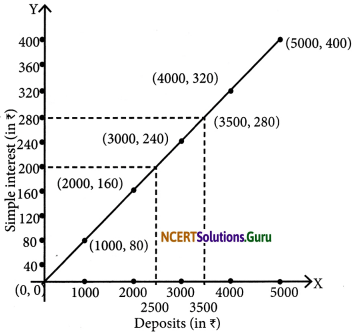(i) Yes, it passes through the origin.
(ii) From the graph, the interest on ₹ 2500 is ₹ 200.
(iii) From the graph, ₹ 3500 should be deposited to get an interest of ₹ 280.

Question 2.
Draw a graph for the following.
(i)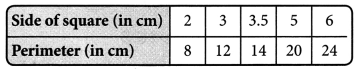Is it a linear graph?
(ii)Is it a linear graph?